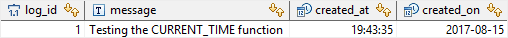# PostgreSQL Tutorial: CURRENT_TIME Function

September 19, 2023

The PostgreSQL `CURRENT_TIME` function returns the current time with time zone.

## Syntax

The following illustrates the syntax of the `CURRENT_TIME` function:

``````CURRENT_TIME(precision)
``````

## Arguments

The `CURRENT_TIME` function accepts one optional argument:

1) `precision`

The `precision` argument specifies the returned fractional seconds precision. If you omit the `precision` argument, the result will include the full available precision.

## Return value

The `CURRENT_TIME` function returns a `TIME WITH TIME ZONE` value that represents the current time with time zone.

## Examples

The following example shows how to get the current time:

``````SELECT CURRENT_TIME;
``````

The output is a `TIME WITH TIME ZONE` value as follows:

``````       timetz
--------------------
19:25:24.805985-07
(1 row)
``````

In this example, we didn’t specify the precision argument, therefore, the full precision available included in the result.

The following example illustrates how to use the `CURRENT_TIME` function with the precision set to 2:

``````SELECT CURRENT_TIME(2);
``````

The result is:

``````     timetz
----------------
19:26:43.01-07
(1 row)
``````

The `CURRENT_TIME` function can be used as the default value of `TIME` columns.

Let’s see the following example.

First, create a table named `log` for the demo:

``````CREATE TABLE log (
log_id SERIAL PRIMARY KEY,
message VARCHAR(255) NOT NULL,
created_at TIME DEFAULT CURRENT_TIME,
created_on DATE DEFAULT CURRENT_DATE
);
``````

The `log` table has the `created_at` column whose default value is the result of the `CURRENT_TIME` function.

Second, insert a row into the `log` table:

``````INSERT INTO log( message )
VALUES('Testing the CURRENT_TIME function');
``````

In the statement, we only specified a value for the `message` column, therefore, other columns got the default values.

Third, check whether the row was inserted into the `log` table with the `created_at` column populated correctly by using the following query:

``````SELECT * FROM log;
``````

The following picture shows the result:As you see, the `created_at` column was populated with the time of which the `INSERT` statement executed.

In this tutorial, you have learned how to use the PostgreSQL `CURRENT_TIME` function to get the current time.